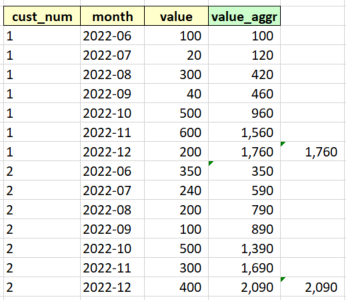# New to Qlik Sense

If you’re new to Qlik Sense, start with this Discussion Board and get up-to-speed quickly.

Announcements
QlikWorld 2023, a live, in-person thrill ride. April 17 - 20, 2023, in Las Vegas! REGISTER TODAY
cancel
Showing results for
Did you mean:Contributor III

## RangeSum(Above) equivalent in Qlik Sense Load Script

I am loading in a file that just has monthly values (fields highlighted in yellow in the below screenshot:

I am trying to return the value being shown in the green highlighted field, which is aggregating based on the month columns (asc).

I can get this to work on the Front End using the RangeSum(Above function

used the set operations in the load script editor, and then in the dashboard, I made the expression as \$(accum) [value] \$(/accum)

In the example below, there are two cust_num's with a month range of 2022-06 - 2022-12.

The accumulated value for 2022-12 should be 1,760 for cust_num 1, and 2,090 cust_num 2

And this is correct as long as all months are showing, and the table is sorted by cust_num, month

But if try to select just one month, it only shows the value for that month. So if you filter for 2022-12, you would not see 1,760, and 2,090, but rather you would see 200, and 400 (Just the value for 2022-12).

https://community.qlik.com/t5/App-Development/Accumulative-Values/td-p/31464Because of this issue, I am trying to do the calculation in the load script. Because Above is not a recognized function in the QlikSense load script editor, the alternative is to use a peek method. So I have tried this:

if(previous("cust_num") = "cust_num", rangesum(peek(value_aggr), (value)),value) as value_aggr

if(previous("month") = "month", rangesum(peek(value_aggr), (value)),value) as value_aggr

if(previous("month","cust_num") = "month", rangesum(peek(value_aggr), (value)),value) as value_aggr

if(previous("month") = "month", if (previous("cust_num") = "cust_num",
rangesum (peek("value_aggr"), "value"), "value"))
as value_aggr

None of the load script have worked as of yet.

Labels (2)

• ### Script

1 Solution

Accepted SolutionsCreator

Have you tried using an order by statement in the table where you do the accumulating? Something like the following:

original_table:

cust_num,

month,

value

from original_source;

noconcatenate

table_with_sum:

*,

if(previous(cust_num) = cust_num, rangesum(peek('value_aggr'), value), value) as value_aggr

resident original_table

order by cust_num, month

;

2 RepliesCreator

Have you tried using an order by statement in the table where you do the accumulating? Something like the following:

original_table:

cust_num,

month,

value

from original_source;

noconcatenate

table_with_sum:

*,

if(previous(cust_num) = cust_num, rangesum(peek('value_aggr'), value), value) as value_aggr

resident original_table

order by cust_num, month

;Contributor III
Author

Thank you for the advice. I think I had a parentheses in the wrong place, which you helped to point out.

I also decided to encase the value_aggr in single quotes.

However neither of those two changes affected the outcome.

But I think what made it work was I needed to have the current field before the previous(field)

vs.

if(previous(cust_num) = "cust_num"

So like below:

if("cust_num" = previous("cust_num"),RangeSum(peek('value_aggr'), value),value) as value_aggr

Thank you for your help on getting me on the right track.

This is where I got the idea to reverse the field comparison